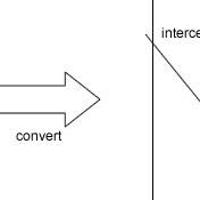## Hough circles in OpenCV

OpenCV comes along with an already made function that detects circles using the hough transform. The Circle Hough Transform is a little inefficient at detecting circles, so it uses the gradient method of detecting circles using the hough transform. Anyway, you don't need to know the details about the internals if you just want to get the command to work.

## The command

The command has the following syntax:

```CvSeq* cvHoughCircles(CvArr* image, void* circle_storage, int method, double dp, double min_dist, double param1=100, double param2=100, int min_radius=0, int max_radius=0);
```

The parameters are similar to that of cvHoughLines2 (Hough transform for lines in OpenCV). I'll go through each parameter in detail:

image is the 8-bit single channel image you want to search for circles in. Because this function uses the gradient method, it automaticall calls cvSobel internally. So, even if you pass a grayscale image, it will automatically generate a binary using cvSobel (internally).

circle_storage is where the function puts its results. You can pass a matrix or a CvMemoryStorage structure here.

method is always `CV_HOUGH_GRADIENT`

dp lets you set the resolution of the accumulator. dp is a kind of scaling down factor. The greater its value, the lower the resolution of the accumulator. dp must always be more than or equal to 1.

min_dist is the minimum distance between circle to be considered different circles.

param1 is used for the (internally called) canny edge detector. The first parameter of the canny is set to param1, and the second is set to param1/2.

param2 sets the minimum number of "votes" that an accumulator cell needs to qualify as a possible circle.

min_radius and max_radius do exactly what to mean. They set the minimum and maximum radii the function searches for.

## Extracting results

To get results, you need to supply a circle_storage. It can be either a matrix of a `CvMemoryStorage` structure.

### CvMat matrix

This is straightforward. You give it a matrix with N rows and 1 column, and in `CV_32FC3` format. It's three channeled to hold the three parameters (x, y and r). In this case, the function returns a NULL.

### CvMemoryStorage memory storage

Here you supply a `CvMemoryStorage` structure, and the function returns a `CvSeq` sequence. You can extract data from thsi sequence like this:

```float* circle = (float*) cvGetSeqElem(circles , index);
```

Then, circle is the x coordinate, circle is the y coordinate and circle is the radius of the circle.

## Done!

This should be enough to get you started with using this function and identifying circles in your images!

#### Related posts#### 7 unique neural network architectures#### Connected Component Labelling#### Converting lines from normal to slope-intercept form

Utkarsh Sinha created AI Shack in 2010 and has since been working on computer vision and related fields. He is currently at Microsoft working on computer vision.